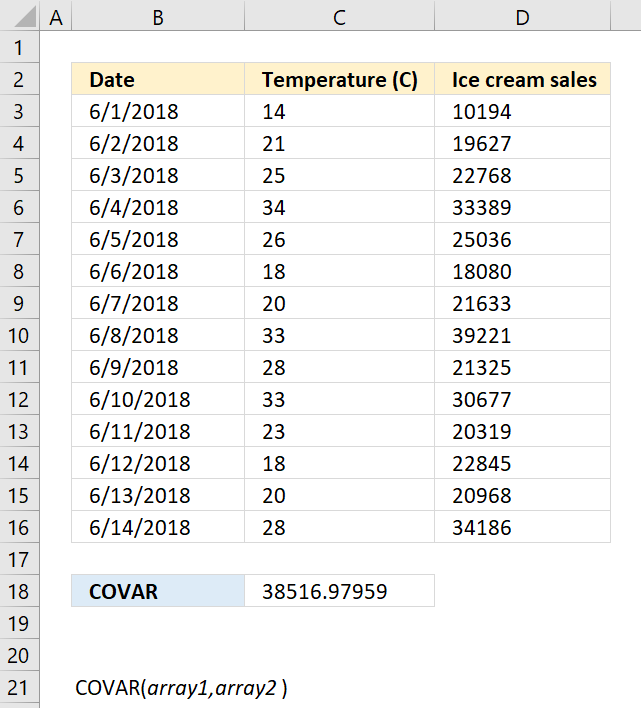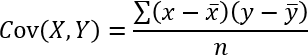Author: Oscar Cronquist Article last updated on October 04, 2018The COVAR function calculates the covariance meaning the average of the products of deviations for each pair in two different datasets. This function is outdated and has been replaced with the COVARIANCE.P and COVARIANCE.S functions.

The covariance is positive if greater values in the first data set correspond to greater values in the second data set. The covariance is negative if greater values in the first data set correspond to smaller values in the second data set.

Formula in cell C18:

=COVAR(C3:C16,D3:D16)

### Excel Function Syntax

COVAR(array1, array2)

### Arguments

 array1 Required. The first data set. array2 Required. The second data set.

Text, logical or empty values are ignored, however, 0 (zeros) are included.

The COVAR function returns

• #N/A error value if the number of data points in array1 and array2 is not equal.
• #DIV/0! error value if either array1 or array2 is empty.

The equation for COVAR is:x ̅  is the sample means AVERAGE(array1) and y ̅  is the sample means AVERAGE(array2).

n is the sample size.